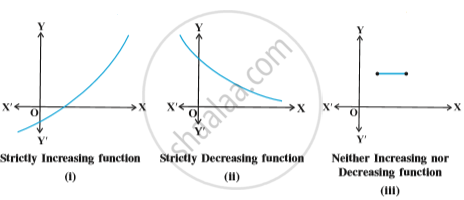Share

# Increasing and Decreasing Functions

#### definition

Let I be an interval contained in the domain of a real valued function f. Then f is said to be
(i) increasing on I if x_1 < x_2 in I ⇒ f(x_1) < f(x_2) for all x_1, x_2 ∈ I.
(ii) decreasing on I, if x_1, x_2 in I ⇒ f(x_1) < f(x_2) for all x_1, x_2 ∈ I.
(iii) constant on I, if f(x) = c for all x ∈ I, where c is a constant.
(iv) decreasing on I if x_1 < x_2 in I ⇒ f(x_1) ≥ f(x_2) for all x_1, x_2 ∈ I.
(v) strictly decreasing on I if x_1 < x_2 in I ⇒ f(x_1) > f(x_2) for all  x_1, x_2 ∈ I.
For graphical representation of such functions see Fig.#### definition

Let x_0 be a point in the domain of definition of a real valued function  f. Then  f  is said to be increasing, decreasing at x_0 if  there exists an open interval I containing x_0 such that f is increasing, decreasing, respectively, in I.

#### theorem

Let  f  be continuous on [a, b] and differentiable on the open interval (a,b). Then
(a) f  is increasing in [a,b] if f′(x) > 0 for each x ∈  (a, b)
(b) f  is decreasing in [a,b] if f′(x) < 0 for each x ∈ (a, b)
(c) f  is a constant function in [a,b] if f′(x) = 0 for each x ∈ (a, b)

Proof:  (a) Let x_1, x_2 ∈ [a, b] be such that x_1 < x_2.
Then, by Mean Value Theorem (Theorem 8 in Chapter 5), there exists a point c between x_1 and x_2 such that
f(x_2) – f(x_1) = f′(c) (x_2 – x_1)
i.e. f(x_2) – f(x_1) > 0        (as f′(c) > 0 (given))

i.e. f(x_2) > f(x_1)

Thus, we have x_1< x_2 f(x_1) f(x_2) ,  for all x_1,x_2 [a,b]

Hence, f  is an increasing function in [a,b].
The proofs of part (b) and (c) are similar. It is left as an exercise to the reader.

#### Video Tutorials

We have provided more than 1 series of video tutorials for some topics to help you get a better understanding of the topic.

Series 1

Series 2

Series 3

### Shaalaa.com

Application of Derivatives part 7 (Increasing, decreasing function) [00:10:44]
S
3
0%

S